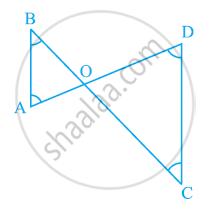Share

# In the given figure, ∠B < ∠A and ∠C < ∠D. Show that AD < BC. - CBSE Class 9 - Mathematics

ConceptInequalities in a Triangle

#### Question

In the given figure, ∠B < ∠A and ∠C < ∠D. Show that AD < BC.#### Solution

In ΔAOB,

∠B < ∠A

⇒ AO < BO (Side opposite to smaller angle is smaller) ... (1)

In ΔCOD,

∠C < ∠D

⇒ OD < OC (Side opposite to smaller angle is smaller) ... (2)

On adding equations (1) and (2), we obtain

AO + OD < BO + OC